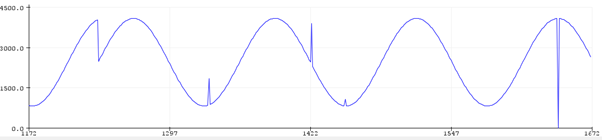# Sinusodial Perameters and SPI drop outs?

Hello!

I have a small project whereby one UNO will send a sine wave to another over SPI. It has mostly gone well and i am receiving the sine wave on the slave side. I have two main issues however, one is that the sinusoidal equation i am using is incorrect for the amplitude or range that i require. I need the maximum value to be 4096 and minimum to be 820 (this is because i plan on using a 12 bit dac some time in the future). I want this to increment on every whole number to give me 3276 total increments, this isnt currently happening in my system. The code for this can be seen below.

``````for(int x=0;x<3276;x++){ // for x 0-3276 increment x, 3276 = total number of potential increments
float y = float (x)/100;
buff = ((sin(y)*1638)+2458); // Min = 820 = 1V, Mid point = 2458 = 3V, Max = 4096 = 5V, Amplitude = 1638

``````

The other issue is that the recieved signal will randomly cut out and show random values at seemingly random intervals as seen in the picture below.I would massively appreciate any help.
Thank you!

My full code is:

``````/* Master */
// SPI Test for 12 BIT DAC

#include <SPI.h>
const int ChipSelect = 10; //SS Pin 10
byte dacPrimaryByte; //Most significant byte
byte dacSecondaryByte; // Least significant byte
uint16_t buff; // 4 zero's + 12 bit value output from Sine wave function

void setup()
{
pinMode (ChipSelect, OUTPUT); // SS Output
digitalWrite(ChipSelect, HIGH); // SS High - Not active
SPI.begin(); // Sets SPI perameters
Serial.begin(9600); // Set baud rate
SPI.setClockDivider(SPI_CLOCK_DIV8); // Slow the clock
}

void loop()
{

for(int x=0;x<3276;x++){ // for x 0-3276 increment x, 3276 = total number of potential increments
float y = float (x)/1000;
buff = ((sin(y)*1638)+2458); // Min = 820 = 1V, Mid point = 2458 = 3V, Max = 4096 = 5V, Amplitude = 1638

// Need to work out the optimum perameters for the above...

byte DACconfig = 0b00110000; // 0011 is most significant 4 bits of PrimaryByte, this will activate the DAC Register, Disable the buffer, Activate gain of 1x, Activate operating mode.
uint16_t dacSecondaryByteMask = 0b0000000011111111; // 8 LSB mask to single out Secondary Byte

byte dacPrimaryByte = byte(buff >> 8) | DACconfig; // Shift 12 bit buff value 8 places leaving 4 bits in the first byte. OR with DACconfig to have 4 config bits and first 4 data bits, 1V (min) = 820, 5v (max) = 4096, 3V (mid) = 2458

byte dacSecondaryByte = byte(buff & dacSecondaryByteMask); // Buff AND Secondary Byte mask leaves only the final 8 bits of Buff. 2 Bytes will be concatenated on slave end

digitalWrite(ChipSelect, LOW); // SS Low = Active
SPI.transfer(dacPrimaryByte); // Transfer most significant byte
SPI.transfer(dacSecondaryByte); // Transfer least significant byte
digitalWrite(ChipSelect, HIGH); // SS High = End of transfer

``````
``````/* Slave */

#include <SPI.h>

byte storage ; // Storage byte for SPI data register
volatile byte pos; // Position within storage byte
volatile boolean process; // Has byte been processed?
uint8_t buff; // Buffer for transfered data
word output_2byte; // 2 Concatenated bytes
word output_12bit; // Concatenated bytes - 4 DAC Config bits
int minout = 819; // Minimum Output_12bit
int maxout = 4096; // Maximum Output_12bit

void setup()
{
pinMode(MISO,OUTPUT);
SPCR |= _BV(SPE); // Enable Slave mode
SPCR |= _BV(SPIE); // Enable interrupts
pos = 0; // Set pos to bit 0
process = false; // Process not yet occured
Serial.begin(9600); // Set baud rate

}

ISR(SPI_STC_vect) // Interrupt service routine
{
byte gathered = SPDR; // Gathered = SPI data register
if( pos < sizeof storage) // If position within storage is smaller than size of storage
{
storage[pos++] = gathered; // Go to next bit in storage byte. Storage byte is equal to SPI data register value
}
else
process = true; // Else the data in SPI data register has been processed
}

void loop()
{
if( process )
{
memcpy(buff,&storage,8); // Copy data from storage to buffer byte array
// Serial.println(buff);
// Serial.println(buff);

output_2byte = word(buff, buff); // Concatenate 2 bytes
output_12bit = output_2byte & 0xFFF; // Remove the first 4 MSB's

Serial.println(output_12bit, '\n'); // Print output and start new line

storage[pos] = 0; // Reset storage
pos = 0; // Reset position
process = false; // Reset process

// delay(90);

}
}
``````

How do You prevent the slave to be overloaded and miss processing?
You can't read from the ISR buffer like that. You must disable interrupt to be sure You get a hole 16 bit int when reading.

This topic was automatically closed 120 days after the last reply. New replies are no longer allowed.